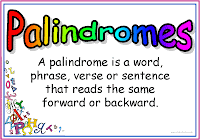## Sunday, November 28, 2010

### C Program For Fibonacci Series

Hello everybody I want to discuss about How to generate a Fibonacci series in C programming.### What is Fibonacci Series?

The first two Fibonacci numbers are 0 and 1 then next number is addition of previous two numbers.

0, 1, 1, 2, 3, 5, 8, 13, 21, 34, 55, 89.....
In mathematics it is defined by recurrence relation.

### Program Code

`  //C Program for generate Fibonacci series#include "stdio.h"#include "conio.h"void main(){ int a,b,c,i,n; clrscr(); a=0; b=1; printf("\n enter n for how many times generate series"); scanf("%d",&n); printf("\n FIBONACCI SERIES\n"); printf("\t%d\t%d",a,b);for(i=0;i<n;i++){     c=a+b;     a=b;     b=c;     printf("\t%d",c); } getch();}`

### Input\Output## Saturday, November 27, 2010

### Digital Clock in C Prgramming

Hello this is program for how to generate a digital clock in c programming.

### Program Code

//simulate a digital clock
#include "stdio.h"
#include "conio.h"
#include "dos.h"
void main()
{
int h,m,s;
h=0;
m=0;
s=0;
while(1)
{

if(s>59)
{
m=m+1;
s=0;
}
if(m>59)
{
h=h+1;
m=0;
}
if(h>11)
{
h=0;
m=0;
s=0;
}
delay(1000);
s=s+1;
clrscr();
printf("\n DIGITAL CLOCK");
printf("\n HOUR:MINUTE:SECOND");
printf("\n%d:%d:%d",h,m,s);
}

}

### Output## Monday, November 15, 2010

### Program Code

// Check whether given number is prime number and not prime number
#include "stdio.h"
#include "conio.h"
void main()
{
int num,i,z;
printf("enter the value of num");
scanf("%d",&num);
i= 2;
while (i less then num)
{
if(num%i==0)
{
z=1;
}
i=i+1;
}
printf("After checking the result is");
if(z== 1)
{
printf("given number is not prime number");
}
else
{
printf("given number is prime number");
}
getch();
}

### Input/Output

enter the value of num 7
After checking the result is
given number is prime number

### What is Prime Number?

Prime number is a natural number which is divisible by 1 and itself.
Example 7

First 25 Prime Number :-
2, 3, 5, 7, 11, 13, 17, 19, 23, 29, 31, 37, 41, 43, 47, 53, 59, 61, 67, 71, 73, 79, 83, 89, 97

## Thursday, November 11, 2010

### C Program to check number is palindrome or not### C Program Code

//Check number is palindrome of not
#include "stdio.h"
#include "conio.h"
void main()
{
int num,rev=0,m,r;
clrscr();
printf("enter any number");
scanf("%d",&num);
m=num;
while(num>0)
{
r=num%10;
rev=rev*10+r;
num=num/10;
}
if(rev==m)
printf("given number is palindrome");
else
printf("given number is not palindrome");
getch();
}

### Input/Output

enter any number 121
given number is palindrome

enter any number 423
given number is not palindrome

### What is Palindrome No?

when we read a number. If backward read is as same as forward read then number is palindrome.## Wednesday, November 10, 2010

### C Program to calculate factorial of given number

You write this code into any c compiler and get factorial of given number by run this code.

C Program Code
//factorial of a number
#include "stdio.h"
#include "conio.h"
void main()
{
int fact=1,n;
clrscr();
printf("enter any number");
scanf("%d",&n);
while(n>0)
{
fact=fact*n;
n--;
}
printf("\n factorial of given number= %d",fact);
getch();
}

Input/Output
enter any number 4
factorial of given number= 24

Factorial of 4 = 4*3*2*1 =24